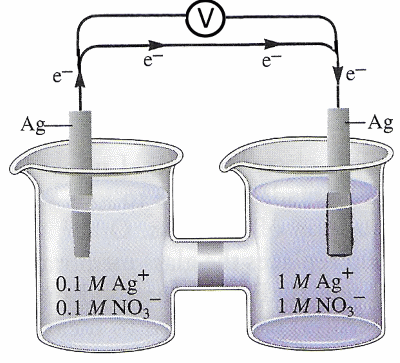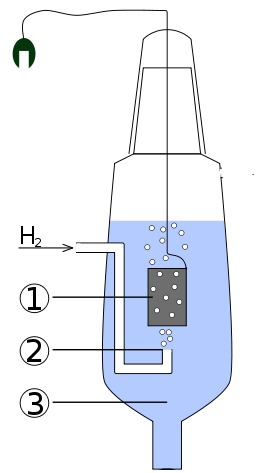Search:

# The oxidation-reduction potential

## The Nernst law### General case: Potential of a couple of oxydo-reduction

Walter Nernst established the law allowing to calculate the oxidation-reduction potential of redox couple in the general case.

For $a\; Oxidant$ $+$ $n\; e^-$ $\rightleftarrows$ $b\; Reductant$ we always have: $E$ $=$ $E^o$ $+$ $\frac{RT}{nF}ln\frac{[Oxidant]^a}{[Reductant]^b}$ with: $E$ : the potential in $Volt$ $E°$: the standard potential in $Volt$ $R$ : the ideal gas constant $R$ $=$ $8.3145 J·mol^{-1}·K^{-1}$ $T$ : the temperature in Kelvin $(K)$ $F$ : Faraday's constant = $96 485 C\cdot mol^{-1}$ $n$ : the number of exchanged electrons $ln$: the natural logarithm with $ln x$ $=$ $2.3log x$ $log$: the logarithm to the base $10$ $[ ]$: molar concentrations (only the dissolved reactants are considered ) in the case of gases, molar concentrations are replaced by partial pressures

In case in which the hydrogen ions are involved, Nernst's equation is modified.

For $a\; Oxidant$ $+$ $c\; H^+$ $+$ $n\; e^-$ $\rightleftarrows$ $b\; Reductant$ $+$ $d\; H_2O$ we always have: $E$ $=$ $E^o$ $+$ $\frac{RT}{nF}ln\frac{[Oxidant]^a[H^+]^c}{[Reductant]^b}$

### In standard conditions:

At $25^oC$, we have: $\frac{RT}{F}$ $=$ $\frac{8.3145\cdot 298.15}{96 485}$ $=$ $0.0256$ and taking into account the conversion between ln and log , Nernst's law becomes:

At $25^oC$ $E$ $=$ $E^o$ $+$ $\frac{0.059}{n}log\frac{[Oxidant]^a}{[Reductant]^b}$ respectively: At $25^oC$: $E$ $=$ $E^o$ $+$ $\frac{0.059}{n}log\frac{[Oxidant]^a[H^+]^c}{[Reductant]^b}$

### Example

The potential of $MnO_4^-$ $+$ $8\; H^+$ $+$ $5\; e^-\;$ $\rightleftarrows$ $Mn^{2+}$ $+$ $4\; H_2O$ in standard conditions is given by $E$ $=$ $1.51$ $+$ $\frac{0.059}{5} log \frac{[MnO_4^-][H^+]^8}{[Mn^{2+}]}$ Taking molar concentrations of 1 $\frac{mol}{L}$ de $MnO_4^-$ and $Mn^{2+}$, we see that the potential varies strongly with the molar concentration of the hydrogen ions (i.e. the acidity):

 $[H^+]$ (in $\frac{mol}{L}$) $E$ (in $V$) $1$ 1.51 $10^{-5}$ 1.04 $10^{-7}$ 0.86

## The electromotive force of a battery

The f.e.m. of a battery is obtained by substracting the potentials of its two electrodes

for instance for the battery $(Zn/Zn^{2+} 0.5\frac{mol}{L})$ || $(Cu/Cu^{2+} 4\frac{mol}{L})$, we have: f.e.m = $E_{Cu/Cu^{2+}}$ $-$ $E_{Zn/Zn^{2+}}$ $=$ $E_{0(Cu/Cu^{2+})}$ $+$ $\frac{0.059}{2} log [Cu^{2+}]$ $-$ $( E_{0(Zn/Zn^{2+})}$ $+$ $\frac{0.059}{2} log [Zn^{2+}])$ $=$ $0.35$ $+$ $\frac{0.059}{2} log\; 4-(-0.76+\frac{0.059}{2} log\; 0.5)$ $=$ $1.14 V$

## Determination of the equilibrium constant of an oxidation-reduction reaction

When the potential difference between the two redox couples in a battery is zero, there is no exchange of electrons. Then the system is in equilibrium and this makes it possible to calculate its equilibrium constant: Sytem 1: $a\; Oxyd._1\;$ $+$ $\;c\; H^+\;$ $+$ $n\; e^-\;$ $\rightleftarrows\;$ $b\; Reduct._1$ $+$ $x\; H_2O$ $E_1$ $=$ $E^o_1$ $+$ $\frac{0.059}{n}log\frac{[Oxyd._1]^a[H^+]^c}{[Reduct._1]^b}$ Sytem 2: $d\; Oxyd._2\;$ $+$ $\;f\; H^+\;$ $+$ $n\; e^-\;$ $\rightleftarrows\;$ $e\; Reduct._2$ $+$ $y\; H_2O$ $E_2$ $=$ $E^o_2$ $+$ $\frac{0.059}{n}log\frac{[Oxyd._2]^d[H^+]^f}{[Reduct._2]^e}$ The equilibrium $a\; Oxyd._1\; + e\; Reduct._2 + (y-x)\; H_2O$ $\rightleftarrows$ $d\; Oxyd._2\; + \;(f-c)\; H^+\; + b\; Reduct._1$ is attained when $E_1$=$E_2$ : $E^o_1-E^o_2$ = $log\frac{[Oxyd._2]^d[H^+]^f}{[Reduct._2]^e}$ $-$ $log\frac{[Oxyd._1]^a[H^+]^c}{[Reduct._1]^b}$ $E^o_1-E^o_2$ = $\frac{0.059}{n}$ · $log\frac{[Oxyd._2]^d[Reduct._1]^b[H^+]^{f-c}}{[Oxyd._1]^a[Reduct._2]^e}$ Here appears the equilibrium constant K of the oxidation-reduction reaction (without $H_2O$)

Equilibrium constant of an oxidation-reduction reaction (without $H_2O$): $log \;K\;=\frac{n}{0.059} E^o$ where $E^o = E^o_1-E^o_2$ is the e.m.f of the battery under standard conditions that can be calculated from the table of redox potentials.

For example, for the reaction $S_4O_6^{2-}+2e^-$ $\rightleftarrows$ $2 S_2O_3^{2-}\; E^o_1= 0.17 \;V$ $Cr^{3+}+e^-$ $\rightleftarrows$ $Cr^{2+}\; E^o_2= -0.50 \;V$ ______________________________ $S_4O_6^{2-}+2Cr^{2+}$ $\rightleftarrows$ $2 S_2O_3^{2-}+2Cr^{3+}$ the equilibrium constant for equilibrium molarities is calculated as follows: $log \;K\;$ $=$ $\frac{[S_2O_3^{2-}]^2[Cr^{3+}]^2}{[S_4O_3^{2-}][Cr^{2+}]^2}$ $=$ $\frac {2\cdot 0.67}{0.059}$ $=$ $22.6$ $K \;=\; 4\cdot 10^{22}\; \frac{mol}{L}$

## Realization of a concentration batteryIn the two compartments of the battery is introduced the same oxidation-reduction couple, but with different concentrations. For example for the battery ($Ag/Ag^{+}$ $0.1\frac{mol}{L}$) || ($Ag/Ag^{+}$ $1\frac{mol}{L}$), we have: $E$ = e.m.f.= $E_{0(Ag/Ag^{+})}$ $+$ $\frac{0.059}{1} log [Ag^{+}]_{left}$ $-$ $( E_{0(Ag/Ag^{+})}$ $+$ $\frac{0.059}{1} log [Ag^{+}]_{right})$ $=$ - $\frac{0.059}{1} log\;\frac{0.10}{1.0}$ $=$ $0.059 V$

## Determination of solubility and solubility product of an ionic substanceIn one of the compartments of the battery is introduced a saturated solution in the presence of the pure substance, in the other a dilute solution of known concentration. For example for the battery ($Ag/Ag^{+}$ $AgI saturé$) || ($Ag/Ag^{+}$ $0.1\frac{mol}{L}$), we measure: $E$ = e.m.f.= $0.417\; V$ By calling $x$ the molarity of $Ag^+$ in the saturated solution, it comes (potential of the battery ) $0.417$ = $E_{0(Ag/Ag^{+})}$ $+$ $\frac{0.059}{1} log [Ag^{+}]_{saturated}$ $-$ $( E_{0(Ag/Ag^{+})}$ $+$ $\frac{0.059}{1} log [Ag^{+}]_{diluted})$ $=$ $0.417$ = $0- 0.059(log\;x-log\;0.100)$ $log\;x$ = $log\;0.100-7.04$ = $-8.04$ $x \;=\; 10^{-8.04}\;=\;9.1\cdot 10^{-9}\;M$ and so: Solubility of $AgI$ = $9.1\cdot 10^{-9}\;M$ Product of solubilty of $AgI$ = $K_{sp}(AgI)$ = $L (AgI)$ = $(9.1\cdot 10^{-9})^2$ = $8.3 \cdot 10^{-17}\;M^2$

## The hydrogen electrode and the determination of the acidityThe electrode (1) is made of platinum which dissolves hydrogen gas (2) provided with a constant pressure $p(H_2)$, the bath is a solution (3) of hydrogen ions $[H^+]$ with a molar concentration of $1 \;\frac{mol}{L}$. Nernst's equation gives the potential of this electrode. For $2 H^+$ $+$ $2 \; e^-$ $\rightleftarrows$ $H_2$ we have : $E$ $=$ $E_0$ $+$ $\frac{0.059}{2} log \frac{[H^+]^2}{p(H_2)}$ Taking into account that $E_0(H^+/H_2)$ $=$ $0\; V$ , we may simplify this equation at atmospheric pressure: $E$ $=$ $0.059\; log [H^+]$ The measure of $E$ at $25^oC$ and $1 \; atm$ allows therefore to determine $[H^+]$ (which mesures the acidity of a solution): $[H^+]$ $=$ $10^{\frac{E}{0.059}}$